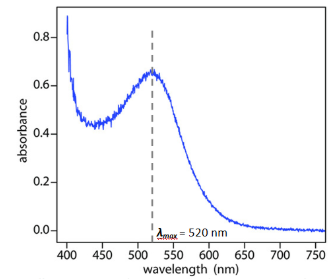# Problem: Spectroscopy is the study of interaction between matter (molecules and atoms) and radiation (e.g. infrared radiation). We use these interactions to obtain information about substances, such as concentration of solutions and the structure of molecules. Different matters interact with radiation differently. The nature of the interaction and the region of the electromagnetic spectrum where these interactions occur depend on the structure of molecules (or atoms). An absorption spectrum is a plot of absorbance versus wavelength, in which the wavelength of maximum absorbance, λmax, is where the absorbance (interaction) is the greatest. For example, as shown in Figure 1, the maximum absorbance (λmax) of the substance is 520 nm.QuestionMatch each dye in the left column with its wavelength of maximum absorbance in the right column.Red dye #40Yellow dye #5   Blue dye #1Answer choices: 628 nm, 504 nm, 427 nm

###### FREE Expert Solution

Recall: ↑ degree of conjugation  = ↑ λmax

The structures of the dye are:

92% (160 ratings)###### Problem Details

Spectroscopy is the study of interaction between matter (molecules and atoms) and radiation (e.g. infrared radiation). We use these interactions to obtain information about substances, such as concentration of solutions and the structure of molecules. Different matters interact with radiation differently. The nature of the interaction and the region of the electromagnetic spectrum where these interactions occur depend on the structure of molecules (or atoms). An absorption spectrum is a plot of absorbance versus wavelength, in which the wavelength of maximum absorbance, λmax, is where the absorbance (interaction) is the greatest. For example, as shown in Figure 1, the maximum absorbance (λmax) of the substance is 520 nm.Question

Match each dye in the left column with its wavelength of maximum absorbance in the right column.

Red dye #40

Yellow dye #5

Blue dye #1

Answer choices: 628 nm, 504 nm, 427 nm

Frequently Asked Questions

What scientific concept do you need to know in order to solve this problem?

Our tutors have indicated that to solve this problem you will need to apply the UV-Vis Spect Basics concept. If you need more UV-Vis Spect Basics practice, you can also practice UV-Vis Spect Basics practice problems.